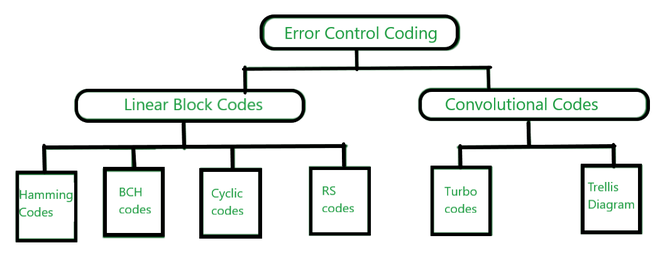# Difference Between Linear Block Codes and Convolutional Codes

Information theory is a branch of probability theory that terms of error probability. An errorless transmission is possible when the probability of error at the receiver approaches 0. It is the numerical computation of the ideas, boundaries, and rules ruling the transmission of messages through communication systems. The information theory is related to the concept of information(entropy), where entropy is the average information of any information source and is denoted by ‘H’.

In a communication system, error-free transmission of the message is the major challenge. Most of the error occurs in the channel or noise. To overcome this issue, error detection, and correction techniques are used.

• Encoding: Encoding is the process of converting a message into the binary form i.e in the form of 0’s and 1’s.
• Decoding: Decoding is the process of extracting the original message from the binary encoded keyword.

This encoding and decoding are used for data transmission from one place to another.

### Linear Block Codes:

It is a simple error control coding technique used for error detection and correction.

• Information data is partitioned into blocks of length K pieces for example Information word.
• Every information word is then coded into a block of length n bits called a codeword. Here, n > k
• Also, n = k + r, where ‘r ‘ denotes the parity bits or check bits added to every information word.
• Vector documentation is utilized for the Data word and Codeword: message m = (m1, m2 mn ), Codeword c = (c1, c2 cn).
• Consists of Hamming codes, Reed-Solomon codes(RS), Bose–Chaudhuri–Hocquenghem(BCH), and cyclic codes.

### Convolutional Codes:

Convolutional code is one more kind of error-correcting code where the resulting bits are acquired by putting out an ideal logical procedure on the present bitstream alongside thinking about the previous bit.

1. In convolutional codes, just the parity bits possibly containing errors are received by the other user or system, which then decodes it to have the most ideal arrangement of bits i.e message bit itself.
2. Shift registers are used to store input bits.
3. Block of ‘n’ digit codeword generated in the encoding depends on not only ‘k’ message digits, but also on the previous m-1 message blocks.
4. Consists of Turbo codes and a Trellis diagram.Error control Coding

### Difference Between Linear Block Codes and Convolutional Codes:

Linear Block codes and Convolutional codes are both error control codes used for error detection and correction coding. The main differences between both these codes are listed below:

All these codes use a different type of algorithm or technique to identify the error bit and to resolve it by adding or removing some bits. Further, there are various types of cyclic codes also for the generation and detection of an error in the channel due to various reasons like noise, etc.

Both the linear block codes and convolutional codes are used in various domains and have a wide range of applications, for example, cybersecurity, cryptography, theory of computation, biology( representing DNA codes), etc.

Unlock the Power of Placement Preparation!
Feeling lost in OS, DBMS, CN, SQL, and DSA chaos? Our Complete Interview Preparation Course is the ultimate guide to conquer placements. Trusted by over 100,000+ geeks, this course is your roadmap to interview triumph.